TitleCollege Algebra
Answer/Discussion to Practice Problems
Tutorial 30B: Operations with FunctionsAnswer/Discussion to 1a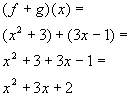*Add the 2 functionsAnswer/Discussion to 1b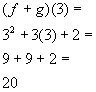*Use the sum found in 1a to plug in 3 for xAnswer/Discussion to 1c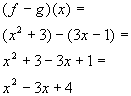*Take the difference of the 2 functions *Subtract EVERY term of the 2nd (   )Answer/Discussion to 1d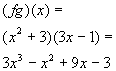*Multiply the 2 functionsAnswer/Discussion to 1e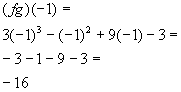*Use the product found in 1d to plug in -1 for xAnswer/Discussion to 1f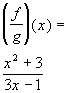*Write as a quotient of the 2 functionsAnswer/Discussion to 1g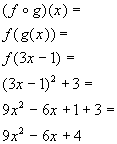*g is inside of f *Substitute 3x - 1 for g *Plug in 3x - 1 for x in function fAnswer/Discussion to 1h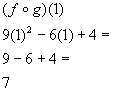*Use the composite function found in 1g to plug in 1 for xAnswer/Discussion to 1i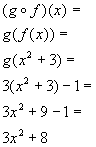*f is inside of g *Substitute x squared + 3 for f *Plug in x squared + 3 for x in function gAnswer/Discussion to 2a   f = {(1, 2), (2, 3), (3, 4), (4, 5)}, g = {(1, -2), (3, -3), (5, -5)}, and h = {(1, 0), (2, 1), (3, 2)}

 When you are looking for the domain of the sum of two functions that are given as sets, you are looking for the intersection of their domains. Since the x values that f and g have in common are 1 and 3, then the domain would be {1, 3}.

 x = 1: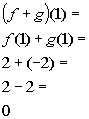(1, 0) *Add together the corresponding y values to x = 1

 x = 3: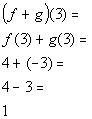(3, 1) *Add together the corresponding y values to x = 3

 Putting it together in ordered pairs we get: f + g = {(1, 0), (3, 1)}Answer/Discussion to 2b    f = {(1, 2), (2, 3), (3, 4), (4, 5)}, g = {(1, -2), (3, -3), (5, -5)}, and h = {(1, 0), (2, 1), (3, 2)}

 When you are looking for the domain of the difference of two functions that are given as sets, you are looking for the intersection of their domains. Since the x values that f and g have in common are 1 and 3, then the domain would be {1, 3}.

 x = 1: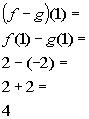(1, 4) *Subtract the corresponding y values to x = 1

 x = 3: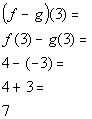(3, 7) *Subtract the corresponding y values to x = 3

 Putting it together in ordered pairs we get: f - g = {(1, 4), (3, 7)}Answer/Discussion to 2c    f = {(1, 2), (2, 3), (3, 4), (4, 5)}, g = {(1, -2), (3, -3), (5, -5)}, and h = {(1, 0), (2, 1), (3, 2)}

 When you are looking for the domain of the product of two functions that are given as sets, you are looking for the intersection of their domains. Since the x values that f and g have in common are 1 and 3, then the domain would be {1, 3}.

 x = 1: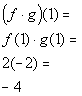(1, -4) *Multiply the corresponding y values to x = 1

 x = 3: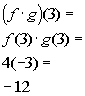(3, -12) *Multiply the corresponding y values to x = 3

 Putting it together in ordered pairs we get: f g = {(1, - 4), (3, -12)}Answer/Discussion to 2d    f = {(1, 2), (2, 3), (3, 4), (4, 5)}, g = {(1, -2), (3, -3), (5, -5)}, and h = {(1, 0), (2, 1), (3, 2)}

 When you are looking for the domain of the quotient of two functions that are given as sets, you are looking for the intersection of their domains AND values of x that do NOT cause the denominator to equal 0. The x values that f and h have in common are 1, 2, and 3.  However, h(1) = 0, which would cause the denominator of the quotient to be 0. So, the domain would be {2, 3}.

 x = 2: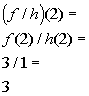(2, 3) *Find the quotient of the corresponding y values to x = 2

 x = 3: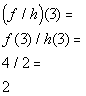(3, 2) *Find the quotient of the corresponding y values to x = 3

 Putting it together in ordered pairs we get: f g = {(2, 3), (3, 2)}Answer/Discussion to 2e  f = {(1, 2), (2, 3), (3, 4), (4, 5)}, g = {(1, -2), (3, -3), (5, -5)}, and h = {(1, 0), (2, 1), (3, 2)}

 When you are looking for the domain of the composition of functions that are given as sets, you are looking for values that come from the domain of the inside function AND when you plug those values of x into the inside function, the output is in the domain of the outside function and so forth. The x values of h are 1, 2, and 3.  However, g(f(h(1))) =g( f(0)), where f(0) is undefined.  Also g(f(h(2))) =g( f(1)) = g(2), where g(2) is undefined.   So, the domain would be {3}.

 x = 3: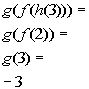(3, -3) *h(3) = 2 *f(2) = 3 *Find the y value that corresponds to x = 3

 Putting it together in an ordered pair we get: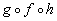= {(3, -3)}Answer/Discussion to 3a

 When you are writing a composition function keep in mind that one function is inside of the other.  You just have to figure out which function is the inside function and which is the outside function. Note that if you put g inside of f you would get: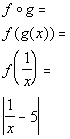*Put g inside of f

 Hey this looks familiar. Our answer is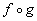.

Last revised on March 31, 2010 by Kim Seward.
All contents copyright (C) 2002 - 2010, WTAMU and Kim Seward. All rights reserved.